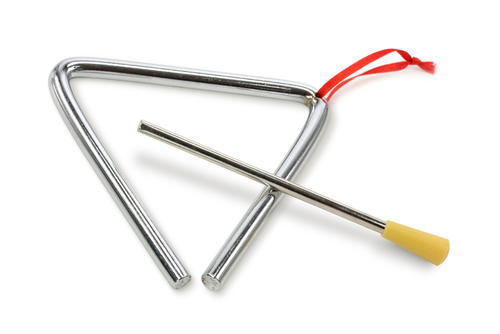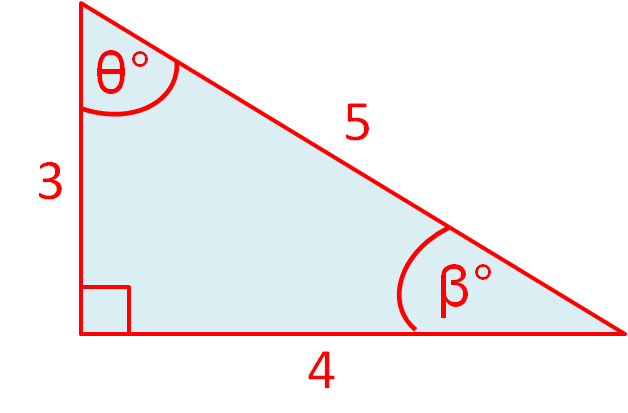• ## Accessibility

Open the accessibility toolbar to change fonts and contrast, access a dictionary, use a ruler and more

Did you play one of these at school when you were younger?The good old days of the triangle before they became bothersome in maths questions.

What examples can you think of that we see in everyday life?Builders may use this a lot for working out areas of gable ends of houses.

You may need the area for cutting patterns in the shape of a triangle to make bunting, or make a kite

Before we can do any of this there is one thing we need to know and that is how to find the area of a triangle.

First we are going back to look at finding the area of a square and rectangle.  Why?  It may help to understand and remember the formula for the area of a triangleArea of a rectangle =base x height

Yes, really that is all there is to it.Look what happens when a diagonal line is drawn from one corner of the rectangle to the other.  By cutting it in half I have made triangles. Cutting it in half is the same as dividing by 2.

Area = base x height ÷ 2

or another way of writing this is

Area = 1/2 base x heightNow you could go barking up the wrong tree, so be careful!

When you are given measurements for a triangle you are sometimes given the base, vertical height and a slanted height.

You always want the vertical height.The area of this triangle  is the base of 4 x vertical height of 3 = 12

12 ÷ 2 = 6 units²

Once you have learned the formula you are flying!

10 questions